# A student

A student is to answer 8 out of 10 questions on the exam.
a) find the number n of ways the student can choose 8 out of 10 questions
b) find n if the student must answer the first three questions
c) How many if he must answer at least 4 of the first 5 questions?

Result

a =  45
b =  21
c =  35

#### Solution:Leave us a comment of example and its solution (i.e. if it is still somewhat unclear...):Be the first to comment!#### To solve this example are needed these knowledge from mathematics:

Would you like to compute count of combinations?

## Next similar examples:

1. Candy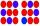How many ways can divide 16 identical candies to 4 children?
2. No. of divisorsHow many different divisors has number ??
3. WordWhat is the probability that a random word composed of chars T, H, A, M will be the MATH?
4. RectanglesHow many rectangles with area 3032 cm2 whose sides is natural numbers are?
5. Seating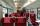How many ways can 6 people sit on 3 numbered chairs (e. G. , seat reservation on the train)?
6. Shelf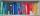How many ways are there to arrange 6 books on a shelf?
7. Phone numbersHow many 7-digit telephone numbers can be compiled from the digits 0,1,2,..,8,9 that no digit is repeated?
8. 7 heroes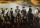9 heroes galloping on 9 horses behind. How many ways can sort them behind?
9. Vans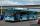In how many ways can 9 shuttle vans line up at the airport?
10. Guests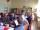How many ways can 5 guests sit down on 6 seats standing in a row?
11. Football league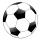In the 5th football league is 10 teams. How many ways can be filled first, second and third place?
12. Playing cards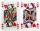How many possible ways are to shuffle 7 playing cards?
13. Pairs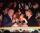At the table sit 8 people, 4 on one side and 4 on the other side. Among them are 3 pairs. Every pair wants to sit opposite each other. How many ways can they sit?
14. One threeWe throw two dice. What is the probability that max one three falls?
15. Flags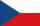How many different flags can be made from colors white, red, green, purple, orange, yellow, blue so that each flag consisted of three different colors?
16. 2nd class variationsFrom how many elements you can create 2450 variations of the second class?
17. Orchard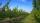3 trees in 3 lines grows in the orchard. How many trees are in the orchard?